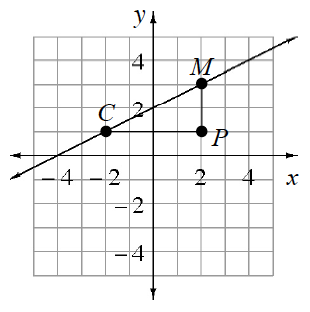### Home > CCG > Chapter 3 > Lesson 3.2.3 > Problem3-77

3-77.

Examine the graph of line $\overleftrightarrow{CM}$ at right.

1. Find the equation of $\overleftrightarrow{CM}$.

You can calculate the slope of the line by using the coordinates of points $C$ and $M$ in the formula below.

$\frac{\Delta y}{\Delta x}=\frac{y_2-y_1}{x_2-x_1}$

2. Find the area and perimeter of $ΔCPM$.

$\text{Area}=4\text{ units}^2$

3. Write an equation of the line through point $M$ that is perpendicular to $\overleftrightarrow{CM}$.

The slopes of perpendicular lines are negative reciprocals.